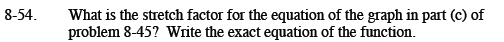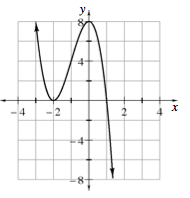Home > CCA2 > Chapter 8 > Lesson 8.1.3 > Problem8-54

8-54.

What is the stretch factor for the equation of the graph in part (c) of problem 8-45? Write the exact equation of the function. Homework Help ✎Using the factors, you can work backwards to find the beginning of the equation. The double root at x = −2 gives you (x + 2)2, the root at x = 1 gives you (x − 1). Substitute the point (0, 8) into the equation y = a(x + 2)2(x − 1) and solve for a.

The stretch factor is −2. The equation is f(x) = −2(x + 2)2(x − 1).